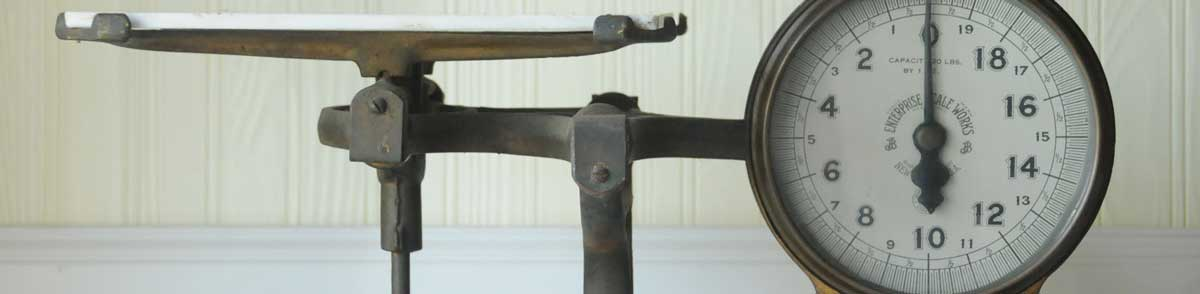# Calories to Grams Conversion

Enter the weight in calories below to get the value converted to grams.

Results in Grams:1 kcal = 0.129598 g
Do you want to convert grams to calories?

## How to Convert Calories to GramsTo convert a calorie measurement to a gram measurement, multiply the weight by the conversion ratio.

Since one calorie is equal to 0.129598 grams, you can use this simple formula to convert:

grams = calories × 0.129598

The weight in grams is equal to the calories multiplied by 0.129598.

For example, here's how to convert 5 calories to grams using the formula above.
5 kcal = (5 × 0.129598) = 0.647989 g

Calories are a unit of energy, and grams are a unit of weight. Keep reading to learn more about each unit of measure.

## Calories

Converting calories is actually a conversion of energy burned by the body performing work rather than actual weight. When measuring the energy content in foods, the large calorie, or kilocalorie, is used, but it's often still just called a "calorie."

One calorie (large calorie) is equal to the heat needed to raise the temperature of one kilogram of water by 1 degree Celsius. You'll need to burn 3,500 calories more than you eat to lose one pound of weight.

Calories can be abbreviated as kcal; for example, 1 calorie can be written as 1 kcal.

## Grams

A gram is the mass/weight equal to 1/1,000 of a kilogram and is roughly equivalent to the mass of one cubic centimeter of water.

The gram, or gramme, is an SI unit of weight in the metric system. Grams can be abbreviated as g; for example, 1 gram can be written as 1 g.

## Calorie to Gram Conversion Table

Calorie measurements converted to grams
Calories Grams
1 kcal 0.129598 g
2 kcal 0.259196 g
3 kcal 0.388793 g
4 kcal 0.518391 g
5 kcal 0.647989 g
6 kcal 0.777587 g
7 kcal 0.907185 g
8 kcal 1.0368 g
9 kcal 1.1664 g
10 kcal 1.296 g
11 kcal 1.4256 g
12 kcal 1.5552 g
13 kcal 1.6848 g
14 kcal 1.8144 g
15 kcal 1.944 g
16 kcal 2.0736 g
17 kcal 2.2032 g
18 kcal 2.3328 g
19 kcal 2.4624 g
20 kcal 2.592 g
21 kcal 2.7216 g
22 kcal 2.8512 g
23 kcal 2.9808 g
24 kcal 3.1103 g
25 kcal 3.2399 g
26 kcal 3.3695 g
27 kcal 3.4991 g
28 kcal 3.6287 g
29 kcal 3.7583 g
30 kcal 3.8879 g
31 kcal 4.0175 g
32 kcal 4.1471 g
33 kcal 4.2767 g
34 kcal 4.4063 g
35 kcal 4.5359 g
36 kcal 4.6655 g
37 kcal 4.7951 g
38 kcal 4.9247 g
39 kcal 5.0543 g
40 kcal 5.1839 g

## References

1. Brazier, Yvette, How many calories do you need?, Medical News Today, https://www.medicalnewstoday.com/articles/263028
2. U.S. Department of Agriculture, I want to lose a pound of weight. How many calories do I need to burn?, https://www.nal.usda.gov/fnic/i-want-lose-pound-weight-how-many-calories-do-i-need-burn